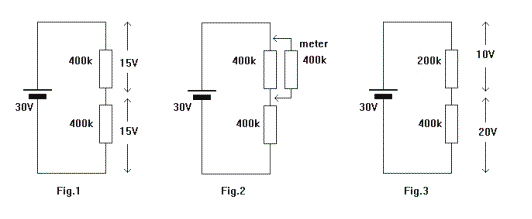Electronic Circuits and Tutorials
Tutorials
Dictionaries
Projects
Discover
Home > Electronics Tutorial > Test and Measurement > Effect of Meter Resistance Tutorial
Test and Measurement in Electronics

# Effect of Meter Resistance Tutorial

All meters have resistance. The value of this resistance depends upon the voltage range selected.

A typical moving coil meter has a SENSITIVITY of 20,000 ohms per volt. This means that when the 1 volt range is selected the meter has a resistance of 20,000 ohms. When the 10 volt range is selected it has a resistance of 200,000 ohms and so on.

When the meter is connected to a circuit to measure voltage, this resistance will affect the circuit and therefore the accuracy of the measurement obtained.In Fig.1 the voltage across each resistor can be calculated.

However, it can be shown that since the resistors are of the same value then the battery voltage divides equally across them, and the voltage across each will be 15 volts.

Now if we set the meter to the 20 volt range to measure this voltage, its resistance will be 20 x 20,000 = 400,000 ohms = 400k. If we connect it across the top resistor, as in Fig.2 then we have two 400k resistors in parallel. Calculating the result of this gives us 200,000 ohms and the circuit looks like Fig.3.

The voltage will now divide to give 10 volts across the top resistor and 20 volts across the lower resistor. The meter will indicate 10 volts when we know that it should indicate 15 volts. Similarly, connecting the meter across the lower resistor will again indicate 10 volts. It appears that there is 10v + 10v = 20 volts across the two resistors, when in fact there is 30 volts.

To obtain the most accurate results, set the meter on the highest range possible. This means that its resistance will be highest and have least effect on the circuit. Digital meter have a very high resistance, typically 10 Megohms on all ranges, and the readings obtained are more accurate than those obtained using a moving coil meter. When buying a new meter look for a sensitivity greater than 20,000 ohms/volt.

Note: To report broken links or to submit your projects, tutorials please email to Webmaster